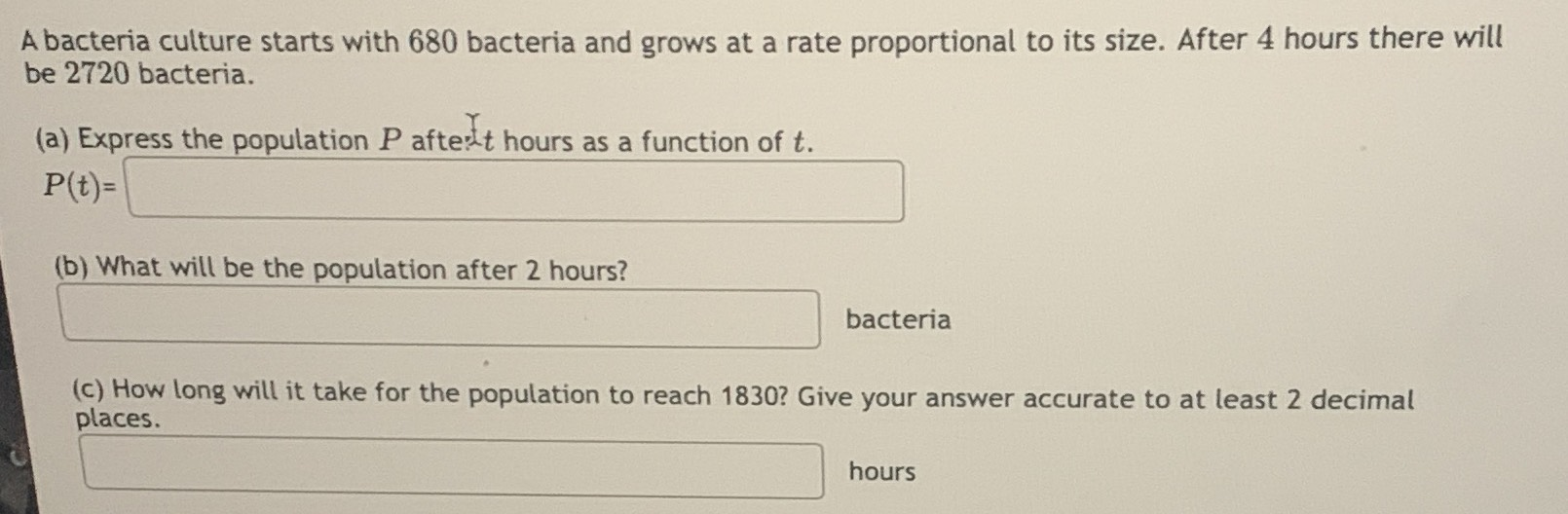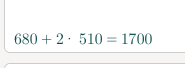### ¿Todavía tienes preguntas de matemáticas?

Pregunte a nuestros tutores expertos
Algebra
PreguntaA bacteria culture starts with $$680$$ bacteria and grows at a rate proportional to its size. After $$4$$ hours there will be $$2720$$ bacteria. (a) Express the population $$P$$ after. $$t$$ hours as a function of $$t$$ .

$$P ( t ) =$$

(b) What will be the population after $$2$$ hours? (c) How long will it take for the population to reach $$1830$$ ? Give your answer accurate to at least $$2$$ decimal places.